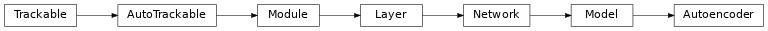# Autoencoder¶

Inheritance Diagramclass ashpy.models.convolutional.autoencoders.Autoencoder(layer_spec_input_res, layer_spec_target_res, kernel_size, initial_filters, filters_cap, encoding_dimension, channels)[source]

Bases: tensorflow.python.keras.engine.training.Model

Primitive Model for all convolutional autoencoders.

Examples

• Direct Usage:

autoencoder = Autoencoder(
layer_spec_input_res=(64, 64),
layer_spec_target_res=(8, 8),
kernel_size=5,
initial_filters=32,
filters_cap=128,
encoding_dimension=100,
channels=3,
)

encoding, reconstruction = autoencoder(tf.zeros((1, 64, 64, 3)))
print(encoding.shape)
print(reconstruction.shape)




Methods

 __init__(layer_spec_input_res, …) Instantiate the BaseAutoEncoder. call(inputs[, training]) Execute the model on input data.

Attributes

 activity_regularizer Optional regularizer function for the output of this layer. dtype dynamic inbound_nodes Deprecated, do NOT use! Only for compatibility with external Keras. input Retrieves the input tensor(s) of a layer. input_mask Retrieves the input mask tensor(s) of a layer. input_shape Retrieves the input shape(s) of a layer. input_spec Gets the network’s input specs. layers losses Losses which are associated with this Layer. metrics Returns the model’s metrics added using compile, add_metric APIs. metrics_names Returns the model’s display labels for all outputs. name Returns the name of this module as passed or determined in the ctor. name_scope Returns a tf.name_scope instance for this class. non_trainable_variables non_trainable_weights outbound_nodes Deprecated, do NOT use! Only for compatibility with external Keras. output Retrieves the output tensor(s) of a layer. output_mask Retrieves the output mask tensor(s) of a layer. output_shape Retrieves the output shape(s) of a layer. run_eagerly Settable attribute indicating whether the model should run eagerly. sample_weights state_updates Returns the updates from all layers that are stateful. stateful submodules Sequence of all sub-modules. trainable trainable_variables Sequence of variables owned by this module and it’s submodules. trainable_weights updates variables Returns the list of all layer variables/weights. weights Returns the list of all layer variables/weights.
__init__(layer_spec_input_res, layer_spec_target_res, kernel_size, initial_filters, filters_cap, encoding_dimension, channels)[source]

Instantiate the BaseAutoEncoder.

Parameters: layer_spec_input_res (tuple of (int, int)) – Shape of the input tensors. layer_spec_target_res – (tuple of (int, int)): Shape of tensor desired as output of _get_layer_spec(). kernel_size (int) – Kernel used by the convolution layers. initial_filters (int) – Numbers of filters to used as a base value. filters_cap (int) – Cap filters to a set amount, in the case of an Encoder is a ceil value AKA the max amount of filters. encoding_dimension (int) – encoding dimension. channels (int) – Number of channels for the reconstructed image. None
call(inputs, training=True)[source]

Execute the model on input data.

Parameters: inputs (tf.Tensor) – Input tensors. training (bool) – Training flag. (encoding, reconstruction) – Pair of tensors.